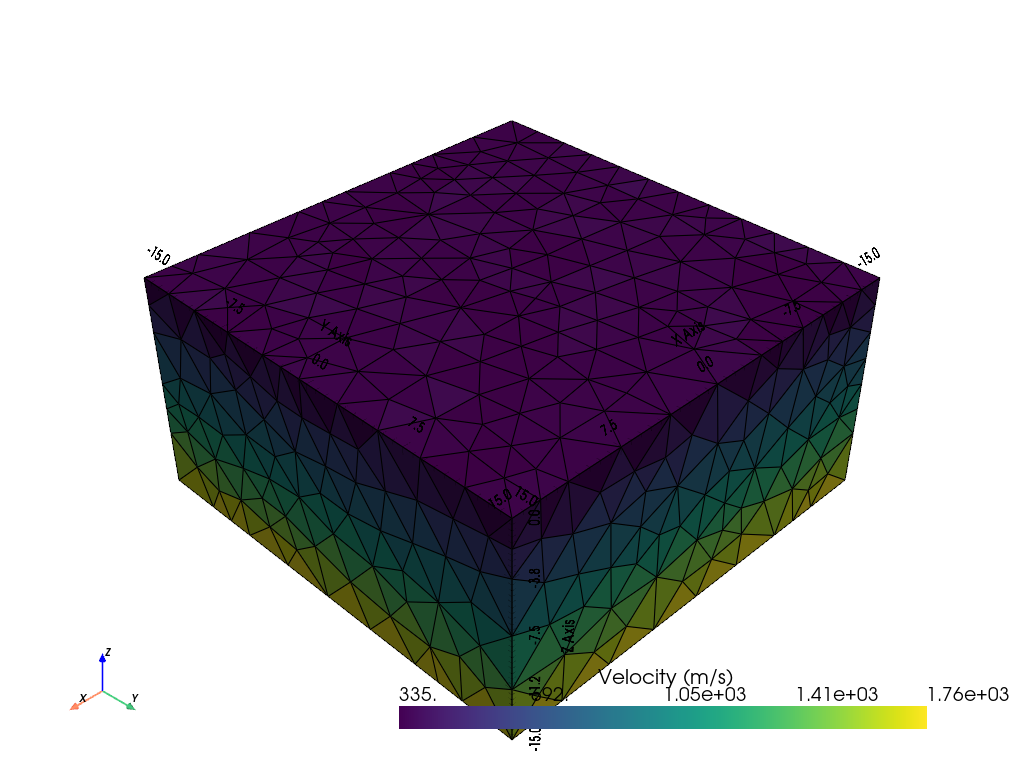# Refraction in 3D#

This example shows refracted ray paths in a three-dimensional vertical gradient medium.

Note

This is a placeholder/proof-of-concept. The code should be refactored partly to tt.showRayPaths()

```import numpy as np
import pygimli as pg
import pygimli.meshtools as mt
from pygimli.physics import traveltime as tt
from pygimli.viewer.pv import drawSensors
import pyvista
```

Build mesh.

```depth = 15
width = 30
plc = mt.createCube(size=[width, width, depth], pos=[0, 0, -depth/2], area=5)

n_sensors = 8
sensors = np.zeros((n_sensors, 3))
sensors[0, 0] = 15
sensors[0, 1] = -10
sensors[1:, 0] = -15
sensors[1:, 1] = np.linspace(-15, 15, n_sensors - 1)

for pos in sensors:
plc.createNode(pos)
mesh = mt.createMesh(plc)
mesh.createSecondaryNodes(1)
```

```vel = 300 + -pg.z(mesh.cellCenters()) * 100
pg.show(mesh, vel, label=pg.utils.unit("vel"), showMesh=True)
``````(<pyvista.plotting.plotter.Plotter object at 0x2adf216c0>, None)
```

Set-up data container.

```data = tt.createRAData(sensors)
data.markInvalid(data("s") > 1)
data.set("t", np.zeros(data.size()))
data.removeInvalid()
```

Do raytracing.

```# fop = pg.core.TravelTimeDijkstraModelling(mesh, data)
fop = tt.TravelTimeModelling()
fop.setData(data)
fop.setMesh(mesh)
print(fop.mesh())

# This is to show single raypaths.
graph = fop.createGraph(1 / vel)
dij = tt.Dijkstra(graph)
dij.setStartNode(mesh.findNearestNode([15, -10, 0]))

rays = []
pos = mesh.positions(withSecNodes=True)[ni]
segs = np.zeros((len(pos), 3))
segs[:, 0] = pg.x(pos)
segs[:, 1] = pg.y(pos)
segs[:, 2] = pg.z(pos)
rays.append(segs)
```
```Mesh: Nodes: 1115 Cells: 4988 Boundaries: 10542 secNodes: 17210
```

Plot final ray paths.

```pl, _ = pg.show(mesh, style='wireframe', line_width=0.1,
hold=True)
drawSensors(pl, sensors, diam=0.5, color='yellow')

for ray in rays:
for i in range(len(ray) - 1):
start = tuple(ray[i])
stop = tuple(ray[i + 1])
line = pyvista.Line(start, stop)```False